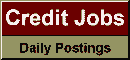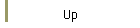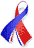DefaultRisk.com the web's biggest credit risk modeling resource.doi> search: A or B Cited by these papersRelated articlesAlternative sources Search RePEc Export citation to:- HTML- Text (plain)- BibTeX- RIS- ReDIF

On Spherical Monte Carlo Simulations for Multivariate Normal Probabilities

by Huei-Wen Teng of National Central University, Taiwan,
Ming-Hsuan Kang of National Chiao Tung University, Taiwan, and
Cheng-Der Fuh of National Central University, Taiwan

September 16, 2013

Abstract: The calculation of multivariate normal probabilities is of great importance in many statistical and economic applications. This paper proposes a spherical Monte Carlo method with both theoretical analysis and numerical simulation. First, the multivariate normal probability is rewritten via an inner radial integral and an outer spherical integral by the spherical transformation. For the outer spherical integral, we apply an integration rule by randomly rotating a predetermined set of well-located points. To find the desired set, we derive an upper bound for the variance of the Monte Carlo estimator and propose a set which is related to the kissing number problem in sphere packings. For the inner radial integral, we employ the idea of antithetic variates and identify certain conditions so that variance reduction is guaranteed. Extensive Monte Carlo experiments on some probabilities calculation confirm these claims.

Keywords: spherical, simulation, variance reduction, kissing number, lattice.

Books Referenced in this paper:  (what is this?)